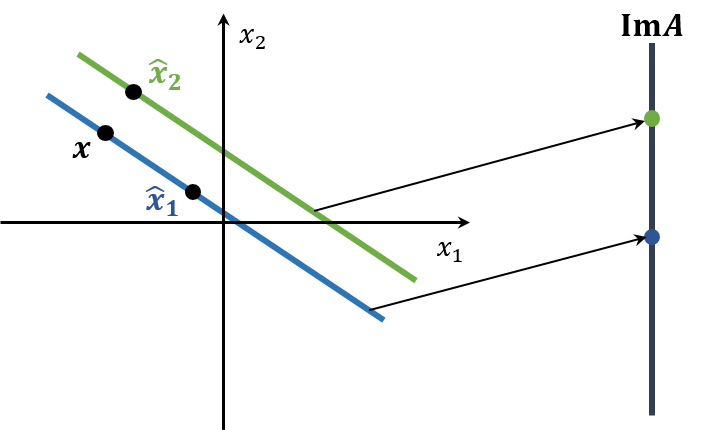# Small Residuals Do Not Imply Small Errors

Consider the following linear system: \begin{aligned} Ax &= \left(\begin{array}{cc} 0.913 & 0.659 \\ 0.457 & 0.330 \end{array}\right) x = \left(\begin{array}{c} 0.254 \\ 0.127 \end{array}\right) = b \end{aligned}

Let the estimated solutions be $\hat{x_1}$ and $\hat{x_2}$, \begin{aligned} \hat{x_1} = \left(\begin{array}{c} -0.0827 \\ 0.5 \end{array}\right), \quad \hat{x_2} = \left(\begin{array}{c} 0.999 \\ -1.001 \end{array}\right) \end{aligned}

and its residuals are \begin{aligned} \left\| r_1 \right\|_1 &= \left\| b - A\hat{x_1} \right\|_1 = 2.1 \times 10^{-4} \\ \left\| r_2 \right\|_1 &= \left\| b - A\hat{x_2} \right\|_1 = 2.4 \times 10^{-2} \\ \end{aligned}

Since $\left\| r_1 \right\| < \left\| r_2 \right\|$, it seems that $\hat{x_1}$ is the optimal solution. Considering the real solution, however, is $x = (1, -1)^t$, it makes more sense that the optimal solution would be $\hat{x_2}$.

This situation happens because $A$ is close to singular. Therefore, when $A$ is ill-conditioned, which means the condition number of $A$ is large ($> 10^4$), this can happen.When $A$ is close to singular, a line in the original space is almost suppresed to a point in the objective space. So, $\hat{x_2}$ which is close to the optimal solution $x$ in the original space may be mapped further than $x$.

## Reference

 Michael T. Heath, Scientific Computing: An Introductory Survey. 2nd Edition, McGraw-Hill Higher Education.